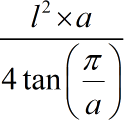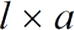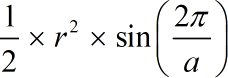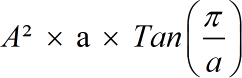Request a Tool

# Area of a regular polygon Calculator

Area of a polygon calculator finds the parameter and area of a regular polygon.

Surface Area
0

#### Formula• π = a constant value 3.1415
• a = Number of sides
• l = Length of the polygon

#### Defination / Uses

Area of a polygon calculator finds the parameter and area of a regular polygon. A Polygon is a closed plane figure having three or more sides. A polygon is a closed planar shape with three or more equal straight sides with all interior angles being equal.

A Polygon is made up of several coplanar line segments that are linked end to end to form a closed shape. Polygons include triangles, rectangles, and pentagons, among others. Regular polygon area calculator also includes the parameter of a polygon calculator.

Perameter
0

#### Formula• a = Number of sides
• l = Length of the polygon

#### Defination / Uses

Area of a polygon calculator finds the parameter and area of a regular polygon. A Polygon is a closed plane figure having three or more sides. A polygon is a closed planar shape with three or more equal straight sides with all interior angles being equal.

A Polygon is made up of several coplanar line segments that are linked end to end to form a closed shape. Polygons include triangles, rectangles, and pentagons, among others. Regular polygon area calculator also includes the parameter of a polygon calculator.

Volume
0

#### Formula• π = a constant value 3.1415
• a = Number of sides
• r = Radius of the polygon

#### Defination / Uses

Area of a polygon calculator finds the parameter and area of a regular polygon. A Polygon is a closed plane figure having three or more sides. A polygon is a closed planar shape with three or more equal straight sides with all interior angles being equal.

A Polygon is made up of several coplanar line segments that are linked end to end to form a closed shape. Polygons include triangles, rectangles, and pentagons, among others. Regular polygon area calculator also includes the parameter of a polygon calculator.

Volume
0

#### Formula• π = a constant value 3.1415
• a = Number of sides
• r = Radius of the polygon
• A = (r) x Cos(π / a)

#### Defination / Uses

Area of a polygon calculator finds the parameter and area of a regular polygon. A Polygon is a closed plane figure having three or more sides. A polygon is a closed planar shape with three or more equal straight sides with all interior angles being equal.

A Polygon is made up of several coplanar line segments that are linked end to end to form a closed shape. Polygons include triangles, rectangles, and pentagons, among others. Regular polygon area calculator also includes the parameter of a polygon calculator.

### How to use polygon calcultor?

There are only two basic steps.

• First select the option from dropdown. What you need to calculate.
• Then add the other values in their corresponding fields.

Thats it! You will get your answer in just a second. use upper given formula in case of manual calculation

The territory occupied by a polygon is referred to as the polygon's area. Regular and irregular polygons exist. Triangles, squares, rectangles, pentagons, hexagons, and other fundamental polygons are utilised in geometry. Each of these polygons has its own region. Because the dimensions of regular polygons are fixed and known to us, it is simple to find the area for them. Because all of the sides of a square are equal, we can simply compute its area if we know the length of one of its sides.